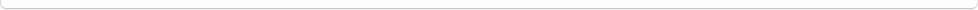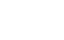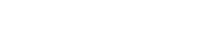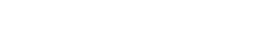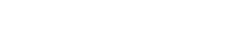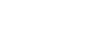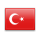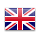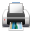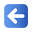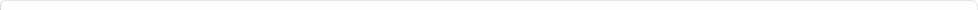Description of Individual Course Units
 Course Unit Code Course Unit Title Type of Course Unit Year of Study Semester Number of ECTS Credits 140400104205 STATISTICS-II Compulsory 2 4 4
Level of Course Unit
First Cycle
Objectives of the Course
This course aims to teach general statistical methods to the students and have them gain basic skills and knowledge about these methods.
Name of Lecturer(s)
Learning Outcomes
 1 Learners can explain the fields in which statistics is used. 2 Learners can define the concepts of statistics. 3 Learners can make compilation and regulation of datas. 4 Learners can explain the characteristics of the normal distribution. 5 Learners can calculate the standard normal curve areas. 6 Learners can calculate the distribution of the sample. 7 Learners can do calculations about the confidence intervals. 8 Learners can explain the basic concepts of hypothesis testing. 9 Learners can do and interpret the results of statistical tests.
Mode of Delivery
Daytime Class
Prerequisites and co-requisities
None
Recommended Optional Programme Components
None
Course Contents
Statistical Definition and basic concepts, data types and methods of data collection, arrangement and presentation of data, measures of central tendency, Measures of Dispersion, Probability theory and random variables, estimation theory
Weekly Detailed Course Contents
 Week Theoretical Practice Laboratory 1 Sampling Theory 2 Distribution of the Sample Means, Distribution of Sample Proportions 3 Sample Distribution of Differences Between Means, Sample Distribution of Differences Between Rates 4 Estimation Theory 5 Point Estimation and Confidence Limits, Confidence Interval for Means, Confidence Intervals for Rates 6 Confidence Intervals for Standard Deviations, Confidence Intervals for Differences Between Means, Confidence Intervals for Differences Between Rates 7 Decision Theory: Basic Concepts 8 Hypothesis Tests for means, ratios, differences between the means. 9 Distribution of Student t and t Test, Confidence Interval for Means, Confidence Interval for Differences Between Means,Hypothesis Tests for averages 10 Chi-Square Test 11 Chi-Square Test 12 Regression and Correlation Analysis 13 Regression and Correlation Analysis 14 Indexes 15 16
Planned Learning Activities and Teaching Methods
Assessment Methods and Criteria
 Term (or Year) Learning Activities Quantity Weight Midterm Examination 1 100 SUM 100 End Of Term (or Year) Learning Activities Quantity Weight Final Examination 1 100 SUM 100 Term (or Year) Learning Activities 40 End Of Term (or Year) Learning Activities 60 SUM 100
Language of Instruction
Turkish
Work Placement(s)
None